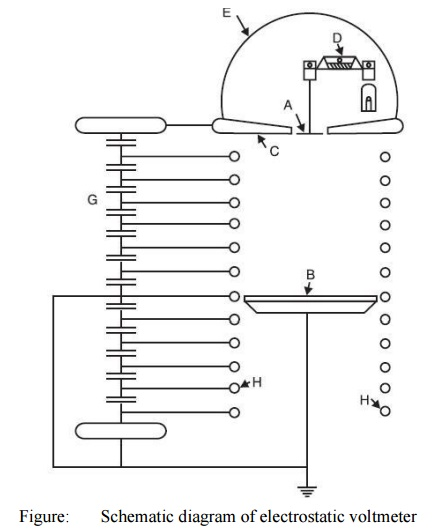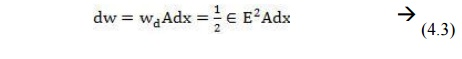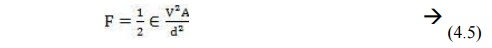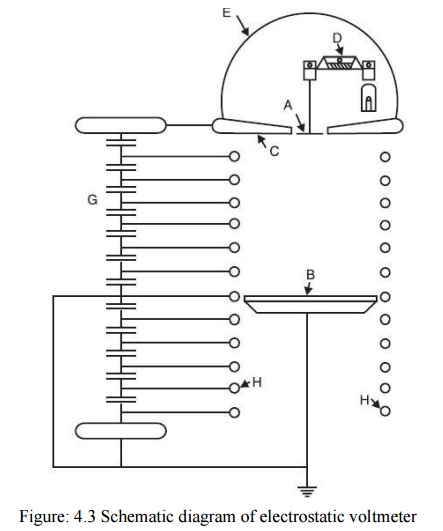Home | | High Voltage Engineering | Electrostatic Voltmeter - Measurement of High Voltage and Currents

# Electrostatic Voltmeter - Measurement of High Voltage and CurrentsThe electric field according to Coulomb is the field of forces. The electric field is produced by voltage and, therefore, if the field force could be measured, the voltage can also be measured.

Electrostatic Voltmeter

The electric field according to Coulomb is the field of forces. The electric field is produced by voltage and, therefore, if the field force could be measured, the voltage can also be measured. Whenever a voltage is applied to a parallel plate electrode arrangement, an electric field is set up between the plates. It is possible to have uniform electric field between the plates with suitable arrangement of the plates. The field is uniform, normal to the two plates and directed towards the negative plate. If A is the area of the plate and E is the electric field intensity between the plates ε the permittivity of the medium between the plates, we know that the energy density of the electric field between the plates is given as,Consider a differential volume between the plates and parallel to the plates with area A and thickness dx, the energy content in this differential volume Adx iNow force F between the plates is defined as the derivative of stored electric energy along the field direction i.e.,Now E = V/d where V is the voltage to be measured and d the distance of separation between the plates. Therefore, the expression for forceSince the two plates are oppositely charged, there is always force of attraction between the plates. If the voltage is time dependant, the force developed is also time dependant. In such a case the mean value of force is used to measure the voltage. ThusElectrostatic voltmeters measure the force based on the above equations and are arranged such that one of the plates is rigidly fixed whereas theother is allowed to move. With this the electric field gets disturbed. For this reason, the movable electrode is allowed to move by not more than a fraction of a millimeter to a few millimeters even for high voltages so that the change in electric field is negligibly small. As the force is proportional to square of Vrms, the meter can be used both for a.c. and d.c voltage measurement.

The force developed between the plates is sufficient to be used to measure the voltage. Various designs of the voltmeter have been developed which differ in the construction of electrode arrangement and in the use of different methods of restoring forces required to balance the electrostatic force of attraction. Some of the methods are

·        Suspension of moving electrode on one arm of a balance.

·        Suspension of the moving electrode on a spring.

·        Pendulous suspension of the moving electrode.

·        Torsional suspension of moving electrode.

The small movement is generally transmitted and amplified by electrical or optical methods. If the electrode movement is minimized and the field distribution can exactly be calculated, the meter can be used for absolute voltage measurement as the calibration can be made in terms of the fundamental quantities of length and force. From the expression for the force, it is clear that for a given voltage to be measured, the higher the force, the greater is the precision that can be obtained with the meter. In order to achieve higher force for a given voltage, the area of the plates should be large, the spacing between the plates (d) should be small and some dielectric medium other than air should be used in between the plates.

If uniformity of electric field is to be maintained an increase in area A must be accompanied by an increase in the area of the surrounding guard ring and of the opposing plate and the electrode may, therefore, become unduly large especially for higher voltages. Similarly the gap length cannot be made very small as this is limited by the breakdown strength of the dielectric medium between the plates. If air is used as the medium, gradients upto5 kV/cm has been found satisfactory. For higher gradients vacuum or SF6gas has been used. The greatest advantage of the electrostatic voltmeter is its extremely low loading effect as only electric fields are required to be set up. Because of high resistance of the medium between the plates, the active power loss is negligibly small. The voltage source loading is, therefore, limited only to their active power required to charge the instrument capacitance which can be as low as a few pico farads for low voltage voltmeters.

The measuring system as such does not put any upper limit on the frequency of supply to be measured. However, as the load inductance and the measuring system capacitance form a series resonance circuit, a limit is imposed on the frequency range. For low range voltmeters, the upper frequency is generally limited to a few MHz. Fig. 4.4 shows a schematic diagram of an absolute electrostatic voltmeter. The hemispherical metal dome D encloses a sensitive balance B which measures the force of attraction between the movable disc which hangs from one of its arms and the lower plate P. The movable electrode M hangs with a clearance of above 0.01 cm, in a central opening in the upper plate which serves as a guard ring. The diameter of each of the plates is 1 metre. Light reflected from a mirror carried by the balance beam serves to magnify its motion and to indicate to the operator at a safe distance when a condition of equilibrium is reached. As the spacing between the two electrodes is large (about 100 cms for a voltage of about 300 kV), the uniformity of the electric field is maintained by the guard rings G which surround the space between the discs M and P. The guard rings G are maintained at a constant potential in space by a capacitance divider ensuring a uniform spatial potential distribution. When voltages in the range10 to 100 kV are measured, the accuracy is of the order of 0.01 per cent. Hueter has used a pair of spheres of 100 cms diameter for the measurement of high voltages utilizing the electrostatic attractive force between them. The spheres are arranged with a vertical axis and at spacing slightly greater than the sparking distance for the particular voltage to be measured. The upper high voltage sphere is supported on a spring and the extension of spring caused by the electrostatic force is magnified by a lamp-mirror scale arrangement. An accuracy of 0.5 per cent has been achieved by the arrangement.Electrostatic voltmeters using compressed gas as the insulating medium have been developed. Here for a given voltage the shorter gap length enables the required uniformity of the field to be maintained with electrodes of smaller size and a more compact system can be evolved. One such voltmeter using SF6 gas has been used which can measure voltages upto 1000 kV and accuracy is of the order of 0.1%. The high voltage electrode and earthed plane provide uniform electric field within the region of a 5 cm diameter disc set in a 65 cm diameter guard plane. A weighing balance arrangement is used to allow a large damping mass. The gap length can be varied between 2.5, 5 and10 cms and due to maximum working electric stress of 100 kV/cm, the voltage ranges can be selected to 250 kV, 500 kV and 100 kV. With 100 kV/cm as gradient, the average force on the disc is found to be0.8681 N equivalent to 88.52 gm wt. The disc movements are kept as small as 1 μm by the weighing balance arrangement. The voltmeters are used for the measurement of high a.c. and d.c voltages. The measurement of voltages lower than about 50 volt is, however, not possible, as the forces become too small.

Study Material, Lecturing Notes, Assignment, Reference, Wiki description explanation, brief detail
High Voltage Engineering : Measurement of High Voltage and High Currents : Electrostatic Voltmeter - Measurement of High Voltage and Currents |